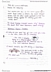# PHYS 131 Midterm: PHYS 131 Midterm Review_Summary (Chapter1-8).pdf

461 views12 pagesamethysteagle405 and 39 others unlocked23
Verified Note
23 documents

## Document Summary

First, choose a team : the newtons, the joules, the watts, the galileans, the hookes. T h e n e wto n s. T h e g alile a n s. Chapter 1: preliminaries: units, significant figures, order of magnitude estimates, dimensional analysis / sanity checks / diagrams. Chapter 2: 1-d kinematics: speed vs velocity / distance vs displacement, average (speed/acceleration) vs instantaneous, graphical approach to disp. , vel. , and acceleration, special case of motion at constant acceleration (eg. freefall): 1 to 8 v = v0 + at vaver = (v + v0)/2 v2 = v0. 2 x = x0 + v0 t + at2. Tells you how fast an object is moving: has a direction that can be determined from two velocity vectors. Is parallel or opposite to the direction of motion. T ells y o u h o w In 1-d motion, the slope at a point on a position-vs-time graph of an object is.